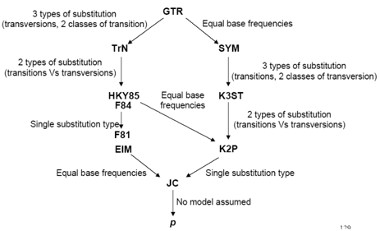### 核苷酸替换模型-GTR是所有模型中考虑参数最多最复杂的模型，其他所有模型是GTR模型的特例

GTR是所有模型中考虑参数最多最复杂的模型，其他所有模型是GTR模型的特例

The use of maximum likelihood (ML) algorithms in developing phylogenetic hypotheses requires a model of evolution. The frequently used General Time Reversible (GTR) family of nested models encompasses 64 models with different combinations of parameters for DNA site substitution. The models are listed here from the least complex to the most parameter rich.

Jukes-Cantor (JC, nst=1): equal base frequencies, all substitutions equally likely (PAUP* rate classification: aaaaaa, PAML: aaaaaa) (Jukes and Cantor 1969)

Felsenstein 1981 (F81, nst=1): variable base frequencies, all substitutions equally likely (PAUP*: aaaaaa, PAML: aaaaaa) (Felsenstein 1981)

Kimura 2-parameter (K80, nst=2): equal base frequencies, one transition rate and one transversion rate (PAUP*: abaaba, PAML: abbbba) (Kimura 1980)

Hasegawa-Kishino-Yano (HKY, nst=2): variable base frequencies, one transition rate and one transversion rate (PAUP*: abaaba, PAML: abbbba) (Hasegawa et. al. 1985)

Tamura-Nei (TrN): variable base frequencies, equal transversion rates, variable transition rates (PAUP*: abaaea, PAML: abbbbf) (Tamura Nei 1993)

Kimura 3-parameter (K3P): variable base frequencies, equal transition rates, two transversion rates (PAUP*: abccba, PAML: abccba) (Kimura 1981)

transition model (TIM): variable base frequencies, variable transition rates, two transversion rates (PAUP*: abccea, PAML: abccbe)

transversion model (TVM): variable base frequencies, variable transversion rates, transition rates equal (PAUP*: abcdbe, PAML: abcdea)

symmetrical model (SYM): equal base frequencies, symmetrical substitution matrix (A to T = T to A) (PAUP*: abcdef, PAML: abcdef) (Zharkikh 1994)

general time reversible (GTR, nst=6): variable base frequencies, symmetrical substitution matrix (PAUP*: abcdef, PAML: abcdef) (e.g., Lanave et al. 1984Tavare 1986Rodriguez et. al. 1990)

In addition to models describing the rates of change from one nucleotide to another, there are models to describe rate variation among sites in a sequence. The following are the two most commonly used models.

gamma distribution (G): gamma distributed rate variation among sites

proportion of invariable sites (I): extent of static, unchanging sites in a dataset

GTR是所有模型中考虑参数最多最复杂的模型，其他所有模型是GTR模型的特例• 发表于 2023-02-02 15:14
• 阅读 ( 152 )
• 分类：基因组学

## 作家榜 »

1.omicsgene 603 文章
2.安生水 280 文章
3.Daitoue 167 文章
4.生物女学霸 120 文章
5.红橙子 78 文章
6.CORNERSTONE 72 文章
7.生信老顽童 53 文章
8.landy 37 文章